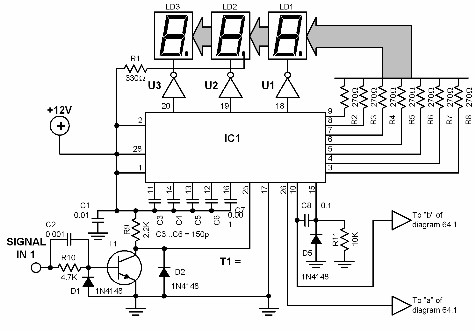# Ratio Meter Circuit Diagrams

Ratio Meter Circuit Diagrams Many times, it is necessary to measure the ratio between two different frequencies, instead of measuring just the absolute value of a single frequency. The ratio meter featured here compares two frequencies, and displays the ratio between them. The higher frequency source is connected to SIGNAL IN 1 (f1) terminal, while the lower frequency source is connected to SIGNAL IN 2 (f2) terminal. The result is displayed digitally by LD1,LD2, and LD3. The measured ratio value that can be displayed is up to 99.9.Ratio Meter Circuit Diagrams

### Ratio Meter Circuit

Many times, it is necessary to measure the ratio between two different frequencies, instead of measuring just the absolute value of a single frequency.

The ratio meter featured here compares two frequencies, and displays the ratio between them.

The higher frequency source is connected to SIGNAL IN 1 (f1) terminal, while the lower frequency source is connected to SIGNAL IN 2 (f2) terminal.

The result is displayed digitally by LD1,LD2, and LD3. The measured ratio value that can be displayed is up to 99.9.

The heart of the circuit is a MK50398 divider IC. The displayed value is exactly the quotient of f1/f2.